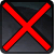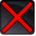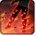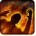# Self Destruct SequenceConditions

## Related effects, buffs and debuffs

Please click on an effect below to view its details.

• [hidden] [20s]Magma Pool

 Slot: Buff Duration: 20s Tick rate: 1s # occurrences: 0 Tags: tag.​abl.​utility.​is_aoe, tag.​abl.​flashpoint.​copero.​boss__bonus.​magma_pool Target overrides: [target override] Aoe Sphere
• When effect ticks
• [target override]

Only when the following conditions are met:

• <
• If PRIMARY TARGET is a player
AND
• If PRIMARY TARGET is alive
>

Perform the following actions:

• Add effect #2 to TARGET from TARGET
• Add effect #3 to TARGET from TARGET
• On Apply

Perform the following actions:

• Play appearance epp . flashpoint . umbara . enemy . boss . tank . cluster_missiles . missile_gtae, dependent on calling effect
• On Apply
• [target override]

Perform the following actions:

• Instantly kill the TARGET
• [3s]Self Destruct Sequence

 Slot: Debuff Duration: 3s Tick rate: does not tick # occurrences: 1 Tags: tag.​abl.​flashpoint.​copero.​boss_bonus.​burning, tag.​abl.​debuff.​damage_over_time, tag.​abl.​dispel_type.​physical, tag.​abl.​flashpoint.​copero.​boss__bonus.​magma_pool Conditions: Can only by called by other effects
• On Apply

Only when the following conditions are met:

• If difficulty is set to 16-man

Perform the following actions:

• Spell Damage
- Unknown (609) = (bool) false
- Damage Type = (int) 3
- Slot = (int) 1
- Spell Type = (int) 2
- Level Cap = (int) 0
- Amount Max = (float) 0
- Amount Min = (float) 0
- Amount Percent = (float) 0
- Threat Percent = (float) 0
- Standard Health Percent Max = (float) 0.08
- Standard Health Percent Min = (float) 0.08
- Amount Modifier Percent = (float) 0
- Coefficient = (float) 0
- Health Steal Percentage = (float) 0
• On Apply

Only when the following conditions are met:

• If difficulty is set to 8-man SM

Perform the following actions:

• Spell Damage
- Unknown (609) = (bool) false
- Damage Type = (int) 3
- Slot = (int) 1
- Spell Type = (int) 2
- Level Cap = (int) 0
- Amount Max = (float) 0
- Amount Min = (float) 0
- Amount Percent = (float) 0
- Threat Percent = (float) 0
- Standard Health Percent Max = (float) 0.08
- Standard Health Percent Min = (float) 0.08
- Amount Modifier Percent = (float) 0
- Coefficient = (float) 0
- Health Steal Percentage = (float) 0
• On Apply

Only when the following conditions are met:

• If difficulty is set to 8-man HM

Perform the following actions:

• Spell Damage
- Unknown (609) = (bool) false
- Damage Type = (int) 3
- Slot = (int) 1
- Spell Type = (int) 2
- Level Cap = (int) 0
- Amount Max = (float) 0
- Amount Min = (float) 0
- Amount Percent = (float) 0
- Threat Percent = (float) 0
- Standard Health Percent Max = (float) 0.08
- Standard Health Percent Min = (float) 0.08
- Amount Modifier Percent = (float) 0
- Coefficient = (float) 0
- Health Steal Percentage = (float) 0
• [4s]Burning
Taking elemental damage over time.

 Slot: Debuff Duration: 4s Tick rate: 1s # occurrences: 1 Tags: tag.​abl.​flashpoint.​copero.​boss_bonus.​burning, tag.​abl.​debuff.​damage_over_time, tag.​abl.​dispel_type.​physical, tag.​abl.​flashpoint.​copero.​boss__bonus.​magma_pool Conditions: Can only by called by other effects
• On Apply

Perform the following actions:

• Play appearance epp . operation . iokath . trash . magma_droid . magma_burst_target_burn, dependent on calling effect
• When effect ticks

Only when the following conditions are met:

• If difficulty is set to 16-man

Perform the following actions:

• Spell Damage
- Unknown (609) = (bool) false
- Damage Type = (int) 3
- Slot = (int) 1
- Spell Type = (int) 2
- Level Cap = (int) 0
- Amount Max = (float) 0
- Amount Min = (float) 0
- Amount Percent = (float) 0
- Threat Percent = (float) 0
- Standard Health Percent Max = (float) 0.04
- Standard Health Percent Min = (float) 0.04
- Amount Modifier Percent = (float) 0
- Coefficient = (float) 0
- Health Steal Percentage = (float) 0
• When effect ticks

Only when the following conditions are met:

• If difficulty is set to 8-man HM

Perform the following actions:

• Spell Damage
- Unknown (609) = (bool) false
- Damage Type = (int) 3
- Slot = (int) 1
- Spell Type = (int) 2
- Level Cap = (int) 0
- Amount Max = (float) 0
- Amount Min = (float) 0
- Amount Percent = (float) 0
- Threat Percent = (float) 0
- Standard Health Percent Max = (float) 0.04
- Standard Health Percent Min = (float) 0.04
- Amount Modifier Percent = (float) 0
- Coefficient = (float) 0
- Health Steal Percentage = (float) 0
• When effect ticks

Only when the following conditions are met:

• If difficulty is set to 8-man SM

Perform the following actions:

• Spell Damage
- Unknown (609) = (bool) false
- Damage Type = (int) 3
- Slot = (int) 1
- Spell Type = (int) 2
- Level Cap = (int) 0
- Amount Max = (float) 0
- Amount Min = (float) 0
- Amount Percent = (float) 0
- Threat Percent = (float) 0
- Standard Health Percent Max = (float) 0.04
- Standard Health Percent Min = (float) 0.04
- Amount Modifier Percent = (float) 0
- Coefficient = (float) 0
- Health Steal Percentage = (float) 0